# 15-344

(diff) ← Older revision | Latest revision (diff) | Newer revision → (diff)

## Introduction to Combinatorics

### Department of Mathematics, University of Toronto, Fall 2015

Agenda: Understand graphs and learn to count.

Instructor: Dror Bar-Natan, drorbn@math.toronto.edu (no math over email!), Bahen 6178, 416-946-5438. Office hours: by appointment.

Classes: Tuesdays 3-5 at MP 202 and Thursdays 2-3 at MP 203.Teaching Assistant: Gaurav Patil (g.patil@mail.utoronto.ca). Office hours: Mondays 3:30-4:30PM at 215 Huron, room 1012, and Tuesdays 6-7PM at math department lounge, on the 6th floor of the Bahen building. Tutorials: Two sessions - Thursdays 4-5 and Thursdays 5-6, both at LM 158.

### Text

Our main text book will be Applied Combinatorics (sixth edition) by Alan Tucker, ISBN 978-0-470-45838-9; it is a required reading.

### Further Resources

 Dror's notes above / Students' notes below

(1). If you took MAT267 (also taught by Professor Bar-Natan), you may remember that Catalan number was discussed in a lecture.

Homework Problems Revisited: Explore the Textbook

(Originally posted in the homework sectors after the corresponding homework was assigned, but moved to here for the readers' convenience.)

(2). For Problem 10, Section 2.3, you may wonder how to untangle the knot without induction. Here is a possible way.

(3). In Problem 85, Section 5.2, we were asked to find the number of triangles formed by pieces of diagonals or outside edges of an n-gon, assuming throughout that no three lines were collinear. What if there is no such constraint? Attached are two papers that presented some general results.

(4).In problem 29, Section 5.3, we were asked to prove that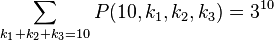$\sum_{k_1 + k_2 + k_3 = 10} P(10, k_1, k_2, k_3) = 3^{10}$, where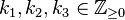$k_1, k_2, k_3 \in \mathbb{Z}_{\geq 0}$.

It is tempting to think that this is a special case of some identity and that a general pattern can be found.

Replacing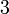$3$ with any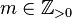$m \in \mathbb{Z}_{>0}$ and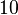$10$ with any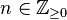$n \in \mathbb{Z}_{\geq0}$ gives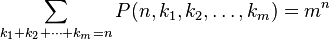$\sum_{k_1+k_2+\cdots+k_m=n} P(n, k_1, k_2, \ldots, k_m) = m^n$

Does it hold, or can it be generalized to a greater extent?

Yes. We have the Multinomial_theorem.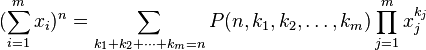$(\sum_{i=1}^m x_i)^n = \sum_{k_1 + k_2 + \cdots + k_m = n} P(n, k_1, k_2, \ldots, k_m) \prod_{j=1}^m x_{j}^{k_{j}}$, where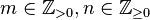$m \in \mathbb{Z}_{>0}, n \in \mathbb{Z}_{\geq0}$ and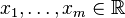$x_1, \ldots, x_m\in \mathbb{R}$.

For a proof by induction, see here.

(5). If you are reading section 7.1 in our textbook, you may find example 3 intriguing. Attempting to solve the problem without looking for a recurrence relation, I found a solution using graph theoretic tools (to be precise, Euler's formula).